# An arc of wire forms a quarter circle whose radius is R relative to the origin....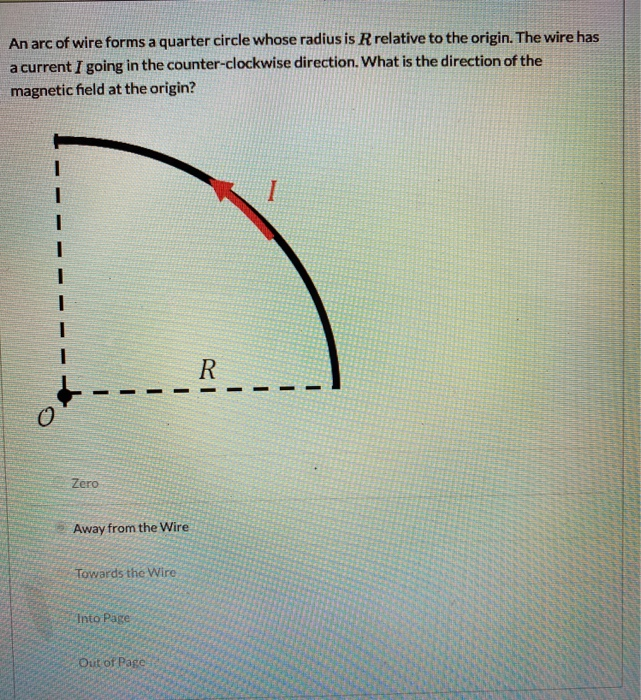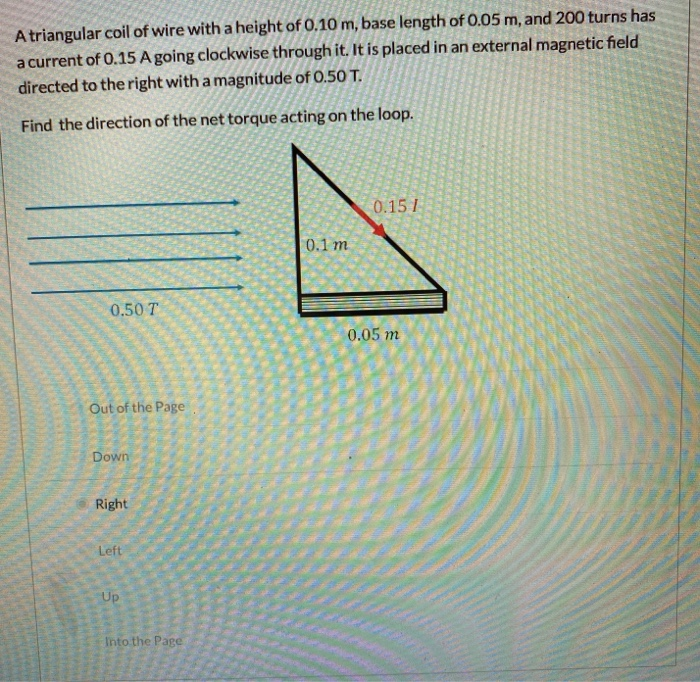An arc of wire forms a quarter circle whose radius is R relative to the origin. The wire has a current I going in the counter-clockwise direction. What is the direction of the magnetic field at the origin? I R - Zero Away from the Wire Towards the Wire Into Page Out of Page
A triangular coil of wire with a height of 0.10 m, base length of 0.05 m, and 200 turns has a current of 0.15 A going clockwise through it. It is placed in an external magnetic field directed to the right with a magnitude of 0.50 T. Find the direction of the net torque acting on the loop. 0.151 0.1 m O 0.50 T 0.05 m Out of the Page Down Right Left Up Into the Page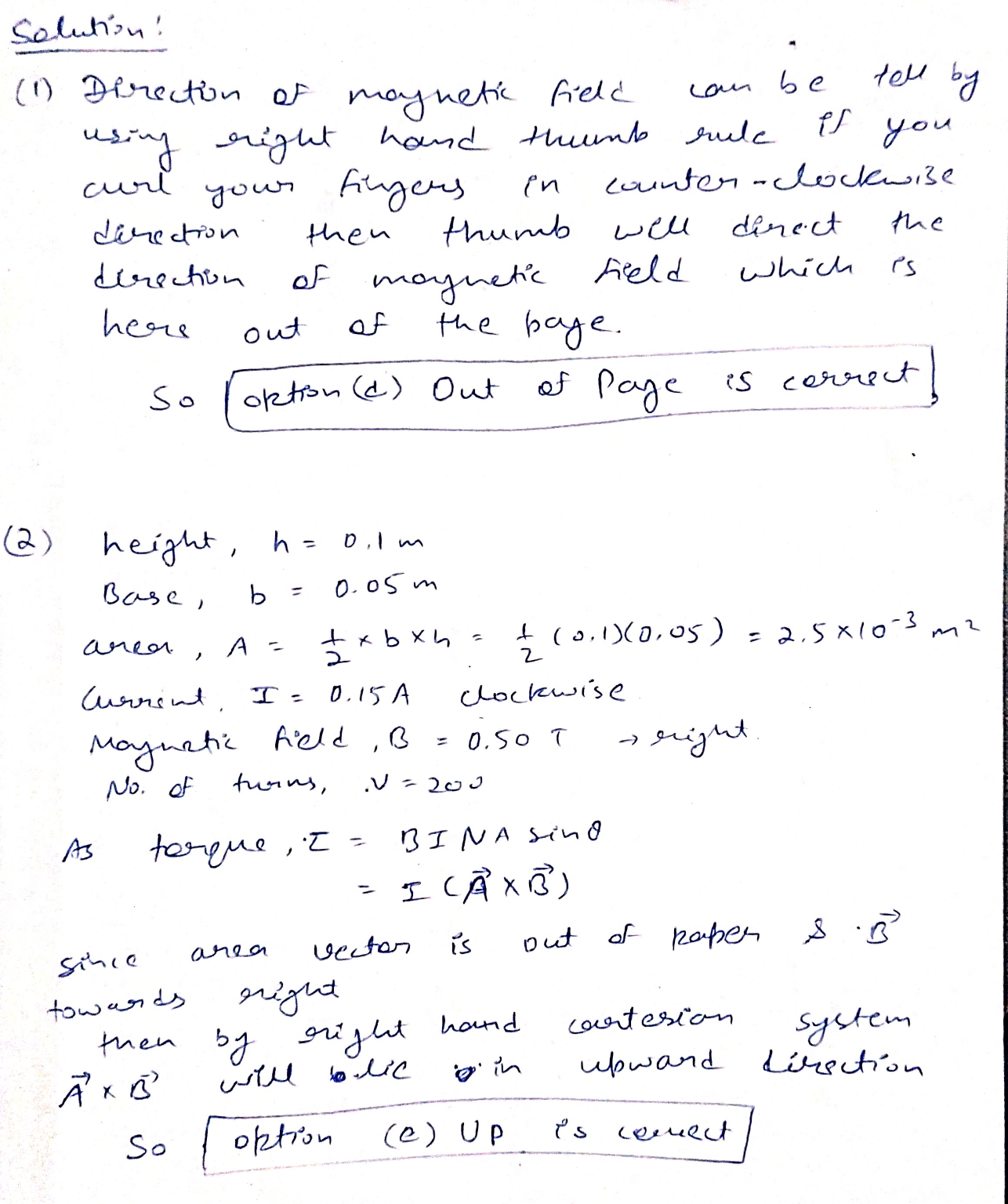I hope it will help you if still having any query related to it then feel free to comment. Plzz rate if u like the answer. Thank you. ?.

##### Add Answer of: An arc of wire forms a quarter circle whose radius is R relative to the origin....
Similar Homework Help Questions
• ### A triangular coil of wire with a height of 0.10 m, base length of 0.05 m,...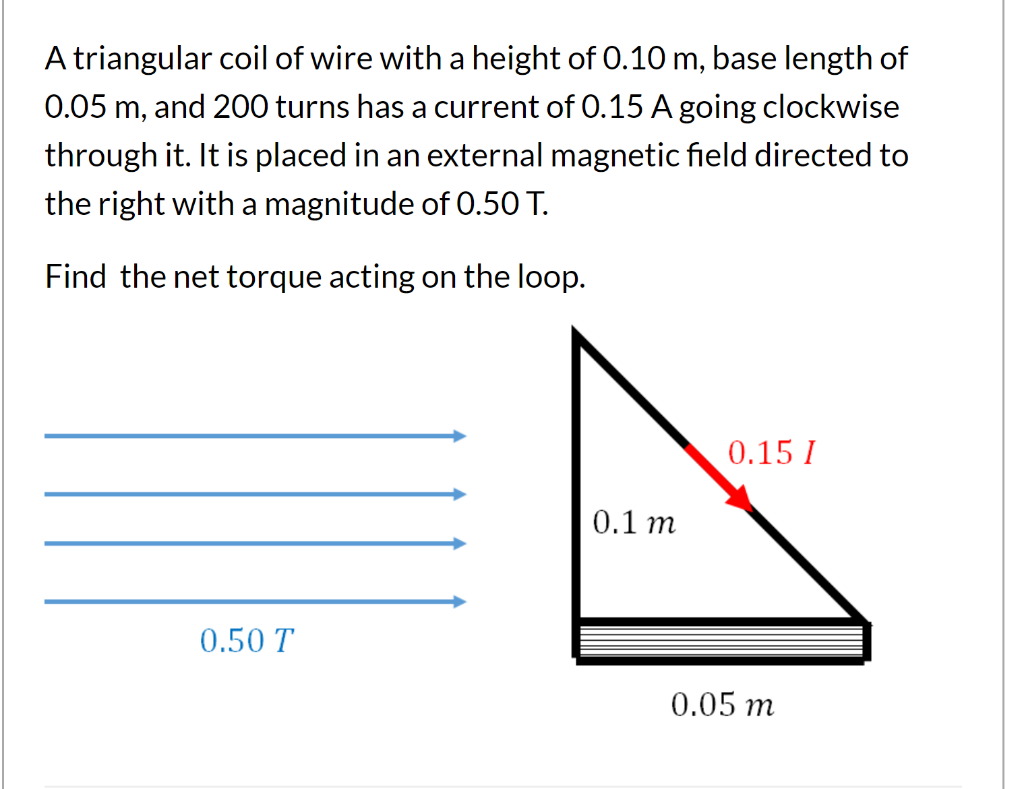A triangular coil of wire with a height of 0.10 m, base length of 0.05 m, and 200 turns has a current of 0.15 A going clockwise through it. It is placed in an external magnetic field directed to the right with a magnitude of 0.50 T. Find the net torque acting on the loop. 0.15 1 0.1 m 0.50 T 0.05 m

• ### Physics II - Direction of Force on a Charge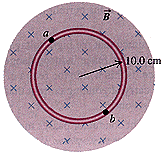Determine the correct direction for the following situations.A. As shown below, a positive charge is moving to the right through a magnetic field that points into the page. Determine the direction of theforce on the charge.Which of the following Directions :ClockwiseleftCounter ClockwiseUpRightInto the PageDownOut of the pageB. As shown below, a negative charge is moving down through a magnetic field that points out of the page. Determine the direction of the force onthe charge.Which of the following Directions :ClockwiseleftCounter ClockwiseUpRightInto...

• ### In the wire shown in segment BC is an arc of a circle with radius 30.0...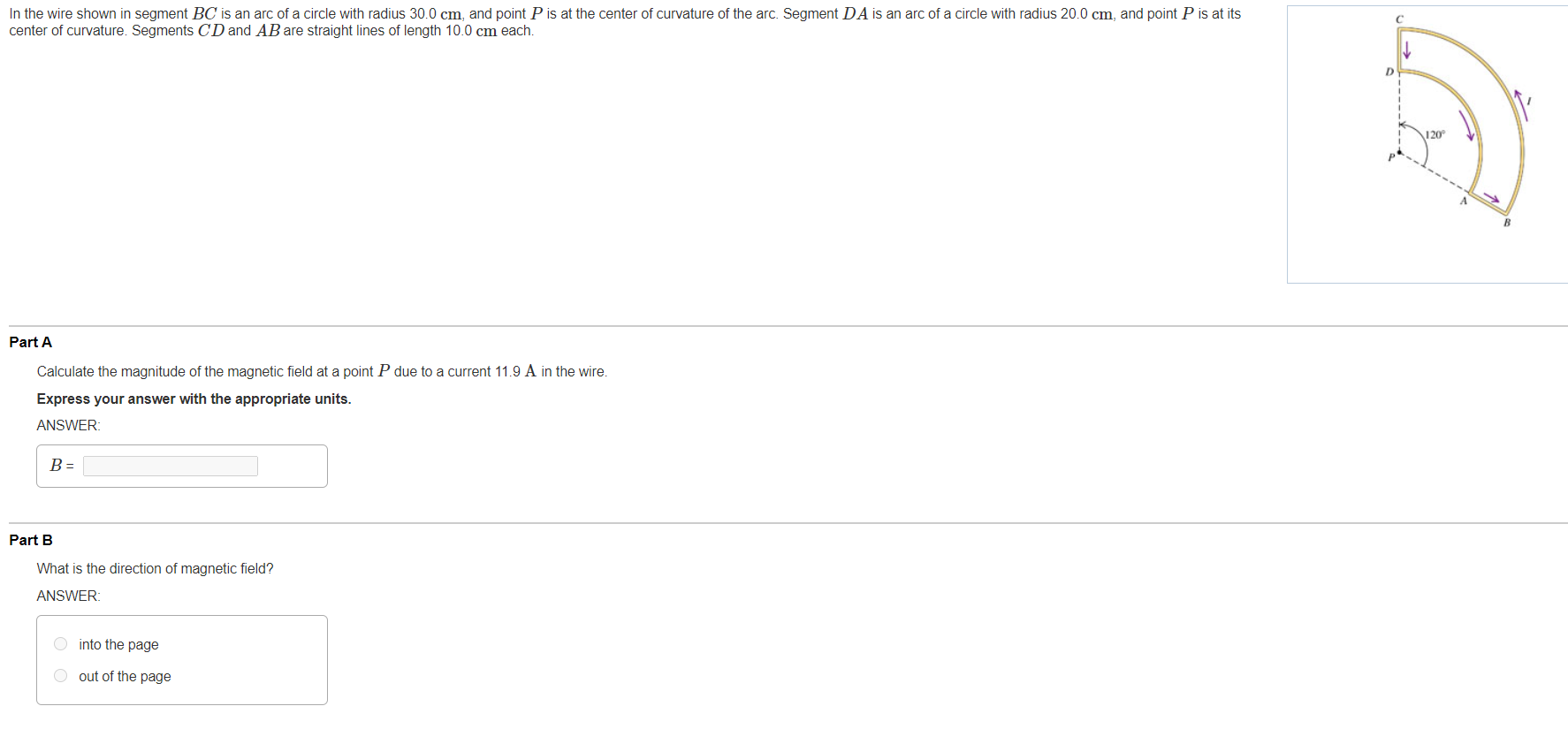In the wire shown in segment BC is an arc of a circle with radius 30.0 cm, and point P is at the center of curvature of the arc. Segment DA is an arc of a circle with radius 20.0 cm, and point P is at its center of curvature. Segments CD and AB are straight lines of length 10.0 cm each. C D 120 B Part A Calculate the magnitude of the magnetic field at a point P due...

• ### The figure below shows a current carrying wire located at the originThe figure below shows a current carrying wire located at the origin; the wire carries a current of I = 4.4 A. Points 1, 2, and 3, are all on a circle with radius15.7 cm which is centered on the origin. (All directions below should be an angle measured counterclockwise from the +x axis to the direction the fieldpoints.)(a) What is themagnitude of the magnetic field generated by the wire at any on the circle??TI For parts (b) - (d),...

• ### The figure below shows a current carrying wire located at the origin. Please help, will rate...Please help, will rate lifesaver! This is a web assign questions, so please give me the right significant digits and units.The figure below shows a current carrying wire located at the origin; the wire carries a current of I = 4.4 A. Points 1, 2, and 3, are all on a circle with radius15.7 cm which is centered on the origin. (All directions below should be an angle measured counterclockwise from the +x axis to the direction the fieldpoints.)(a) What...

• ### The rectangular coil in the figure below has 94 turns, is 25 cm wide and 30...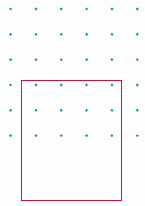The rectangular coil in the figure below has 94 turns, is 25 cm wide and 30 cm long, and is located in a magnetic field B = 0.14 T directed out of the page as shown. Only half of the coil in the region of the magnetic field. The resistance of the coil is 23 ?. Find the magnitude and direction of the induced current if the coil is moving with a velocity of 2.0 m/s to the right, up...

• ### 4.A long, straight wire carrying a current moving upward is placed in a magnetic field produced...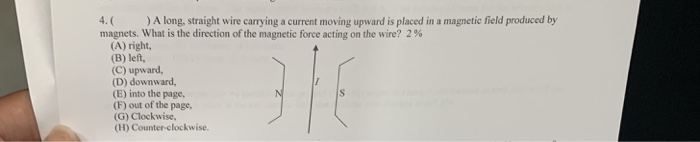4.A long, straight wire carrying a current moving upward is placed in a magnetic field produced by magnets, what is the direction of the magnetic force acting on the wire? 2 % (A) right, (B) left, (C) upward, (D) downward, (E) into the page, (F) out of the page, (G) Clockwise (H) Counter-clockwise.

• ### PRACTICE IT Use the worked example above to help you solve this problem. A circular wire...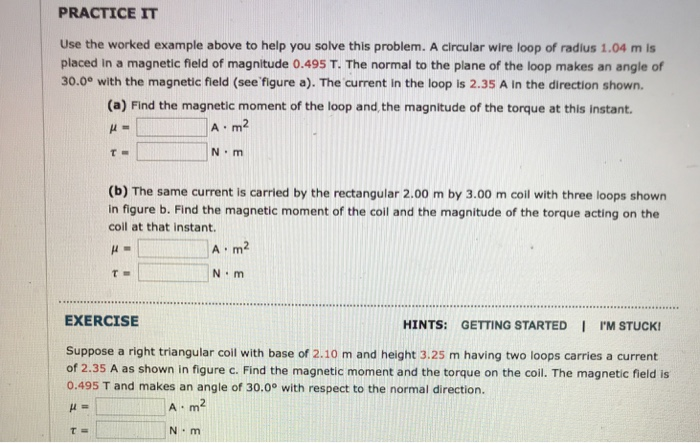PRACTICE IT Use the worked example above to help you solve this problem. A circular wire loop of radius 1.04 m is placed in a magnetic field of magnitude 0.495 T. The normal to the plane of the loop makes an angle of 30.0e with the magnetic field (see figure a). The current in the loop is 2.35 A in the direction shown. (a) Find the magnetic moment of the loop and,the magnitude of the torque at this instant. A...

• ### A current-carrying wire moves toward a coil A long straight wire carrying current I is moving...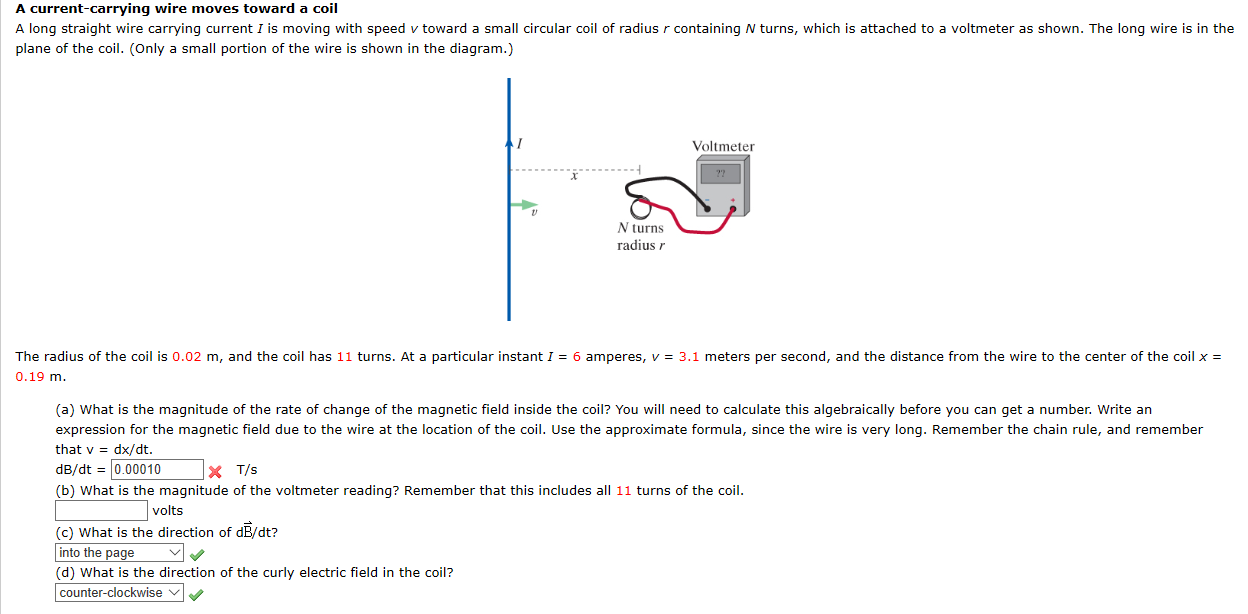A current-carrying wire moves toward a coil A long straight wire carrying current I is moving with speed v toward a small circular coil of radius r containing N turns, which is attached to a voltmeter as shown. The long wire is in the plane of the coil. (Only a small portion of the wire is shown in the diagram.) Voltmeter N turns radius r The radius of the coil is 0.02 m, and the coil has 11 turns. At...

• ### Q11) A circular loop of wire with radius r= 0.0480m and reistance R 0.160 ? is...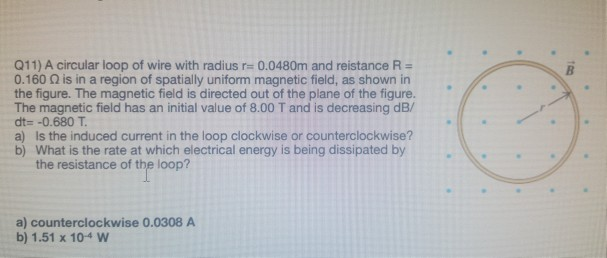Q11) A circular loop of wire with radius r= 0.0480m and reistance R 0.160 ? is in a region of spatially uniform magnetic field, as shown in the figure. The magnetic field is directed out of the plane of the figure. The magnetic field has an initial value of 8.00 T and is decreasing dB/ dt- -0.680 T a) Is the induced current in the loop clockwise or counterclockwise? b) What is the rate at which electrical energy is being...

Free Homework App